Gradient descent with large data, stochastic gradient descent, mini-batch gradient descent, map reduce, data parallelism, and online learning.

## 1. Gradient Descent with Large Data Sets

I would like to give full credits to the respective authors as these are my personal python notebooks taken from deep learning courses from Andrew Ng, Data School and Udemy :) This is a simple python notebook hosted generously through Github Pages that is on my main personal notes repository on https://github.com/ritchieng/ritchieng.github.io. They are meant for my personal review but I have open-source my repository of personal notes as a lot of people found it useful.

### 1a. Learning with Large Data Sets

• Why do we want large data set?
• This is evident when we take a low-bias learning algorithm and train it on a lot of data
• The example of how “I ate two (two) eggs” shows how the algorithm performs well when we feed it a lot of data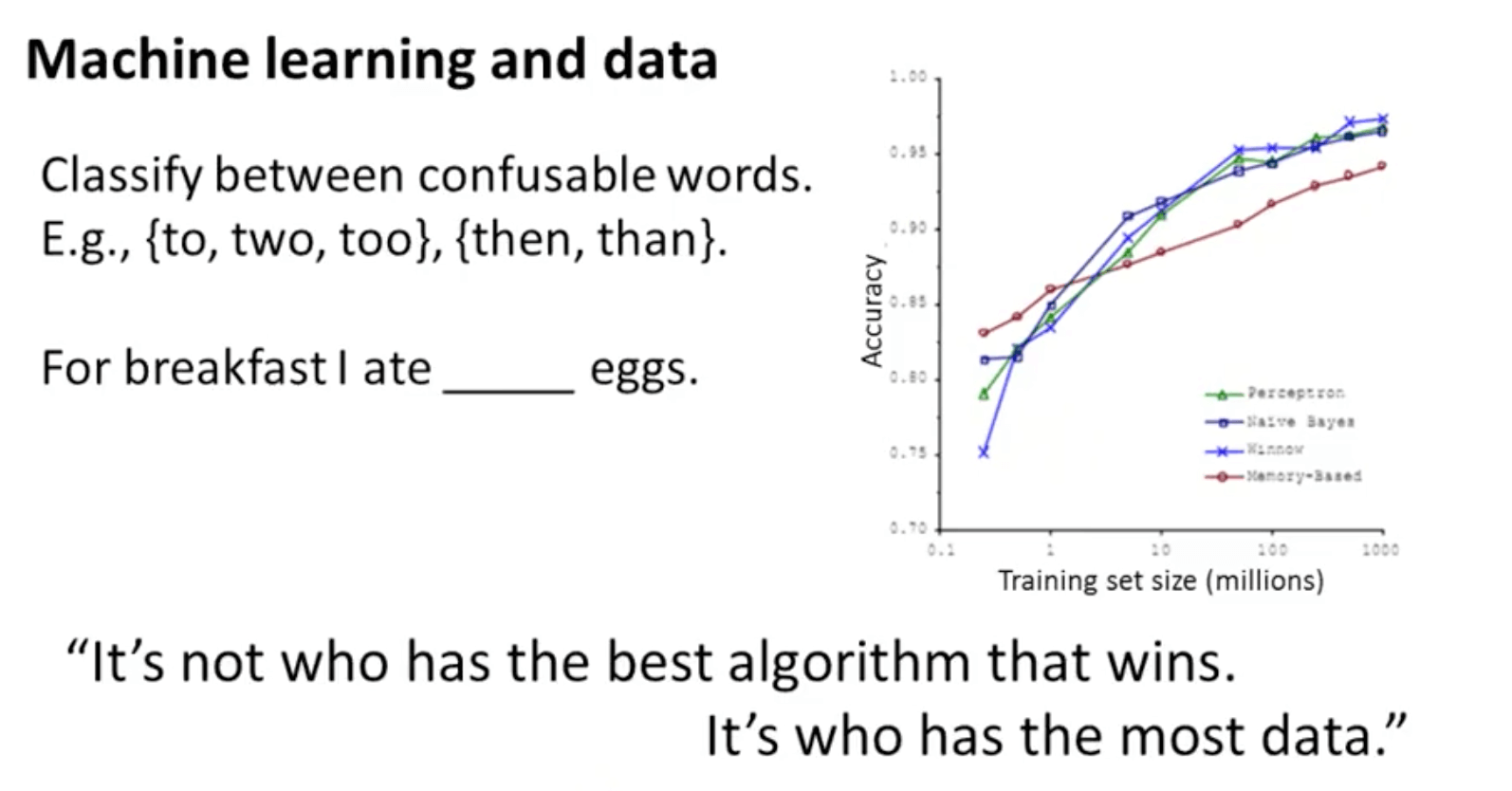• Learning with large data sets has computational problems
• If m = 100m, we have to sum over 100m entries to compute one step of gradient descent
• Suppose you are facing a supervised learning problem and have a very large data set (m = 100m), how can you tell if the data is likely to perform much better than using a small subset (m = 100) of the data?
• Plot a learning curve for a range of values of m and verify that the algorithm has high variance when m is small
• We would then be confident that adding more training examples would increase the accuracy
• But if it’s high bias, we do not need to plot to a large value of m
• In this case, you can add extra features or units (in neural networks)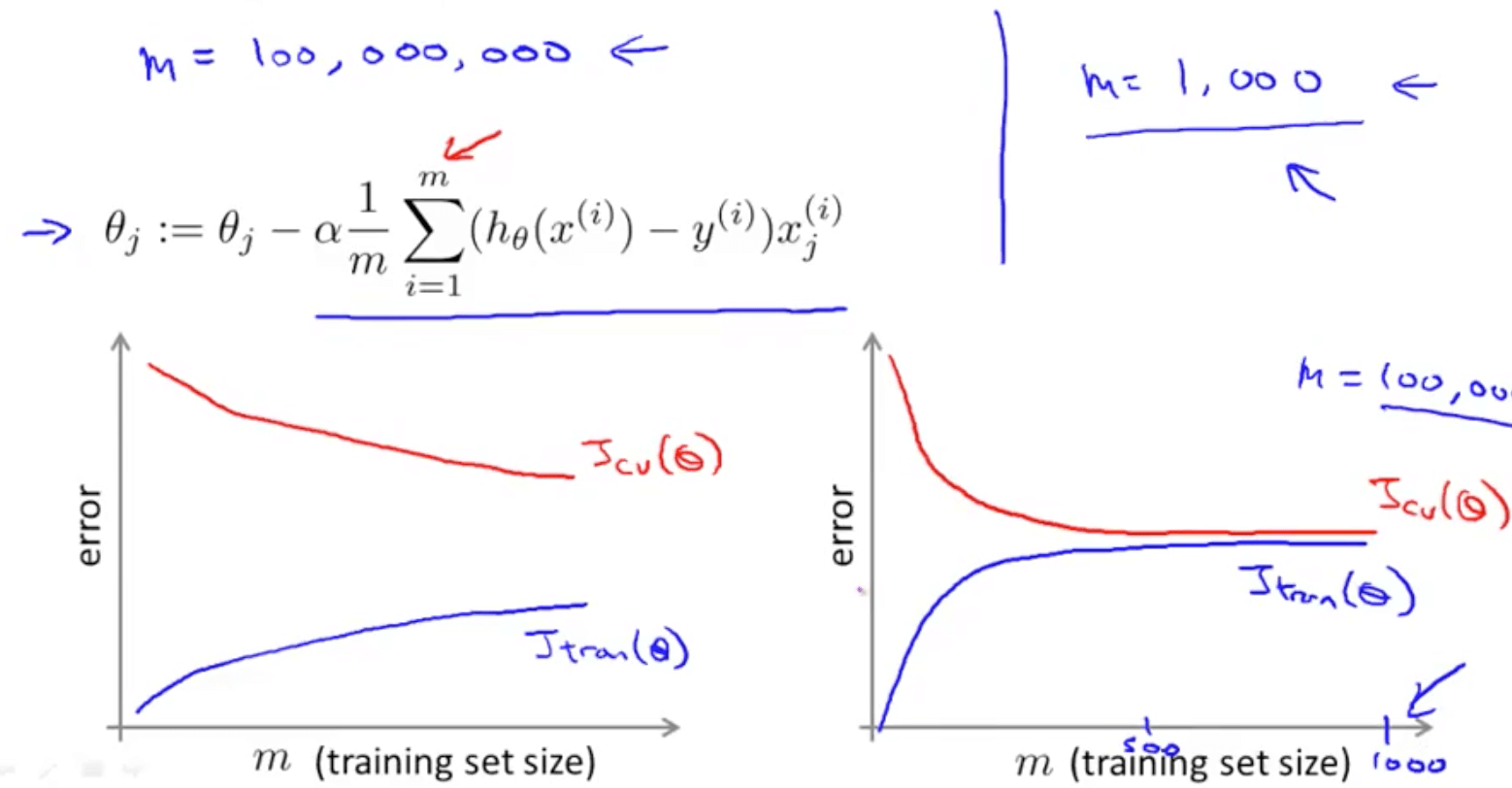• Suppose we are training a linear regression model with gradient descent
• If m is really large, we have to sum across all the examples
• This is actually called batch gradient descent when you look at all the training examples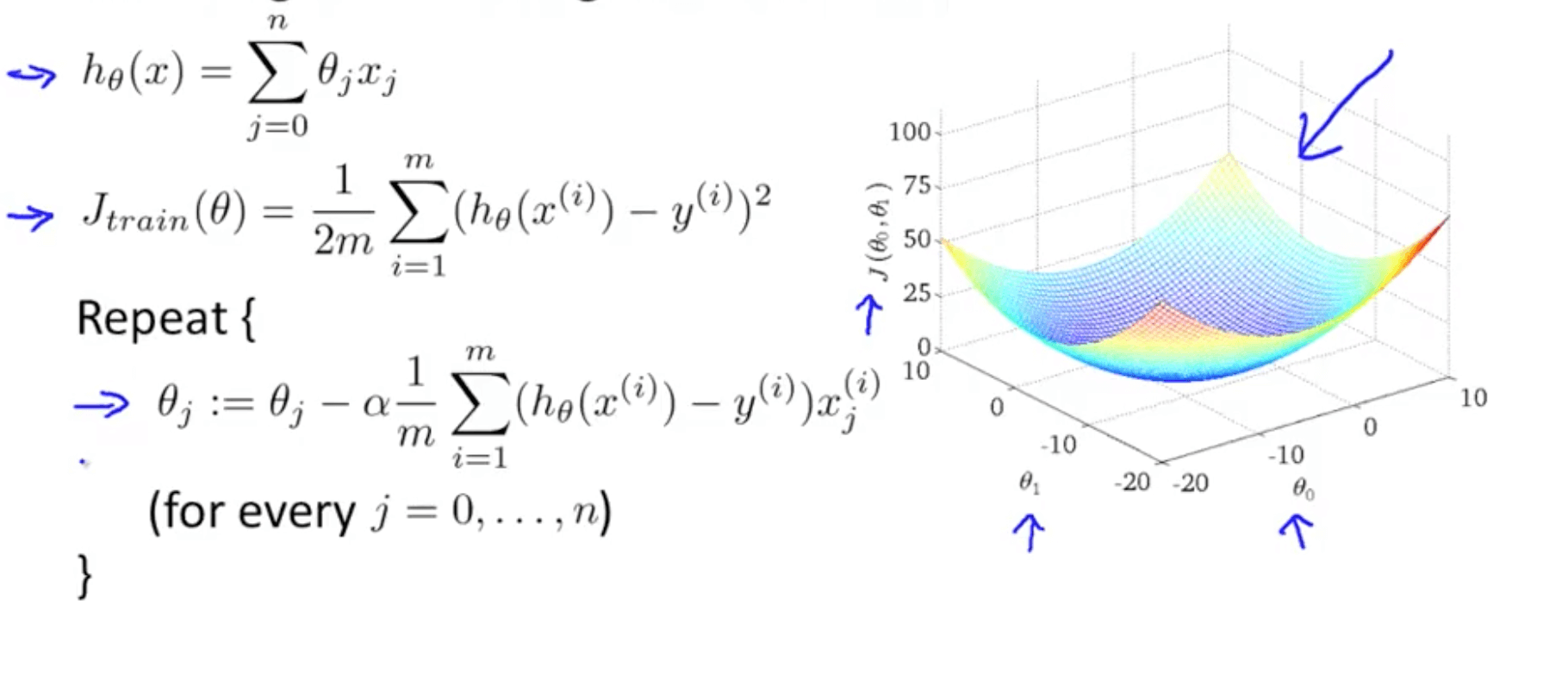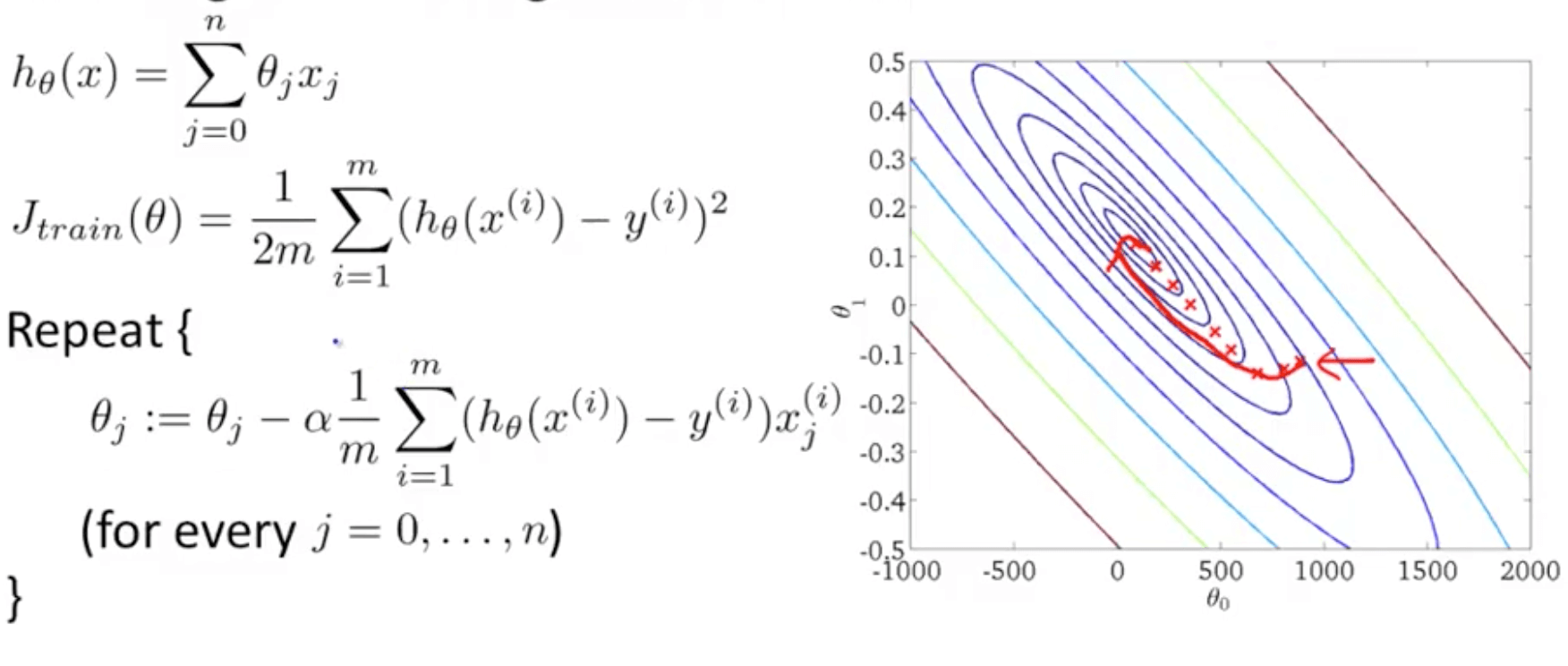• Stochastic gradient descent (more efficient)
• Define cost of parameter θ with respect to x_i and y_i
• This measures how well the hypothesis is doing on a single training example (x_i, y_i)
• This algorithm will take the first training example and take a step (modify parameter) to fit the first example better until the end of the training set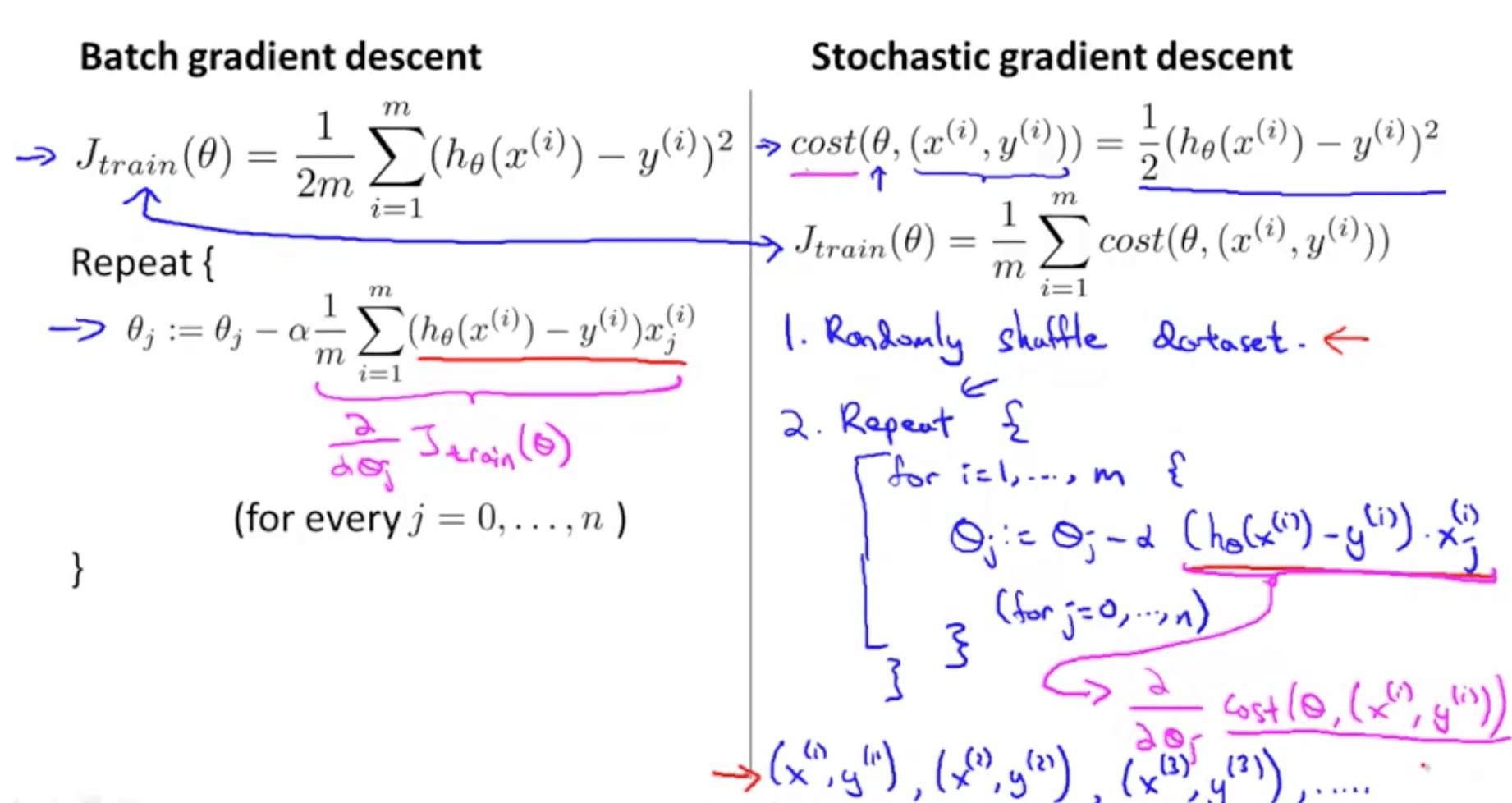• Difference
• Rather than waiting to take the parts of all the training examples (batch gradient descent), we look at a single training example and we are making progress towards moving to the global minimum
• Batch gradient descent (red path)
• Stochastic gradient descent (magenta path with a more random-looking path where it wonders around near the global minimum)
• In practice, so long the parameters are near the global minimum, it’s sufficient
• We can repeat the loop maybe 1 to 10 times
• It is possible even with 1 loop, where your m is large, you can have good parameters
• Your J_train (cost function) may not decrease with every iteration for stochastic gradient descent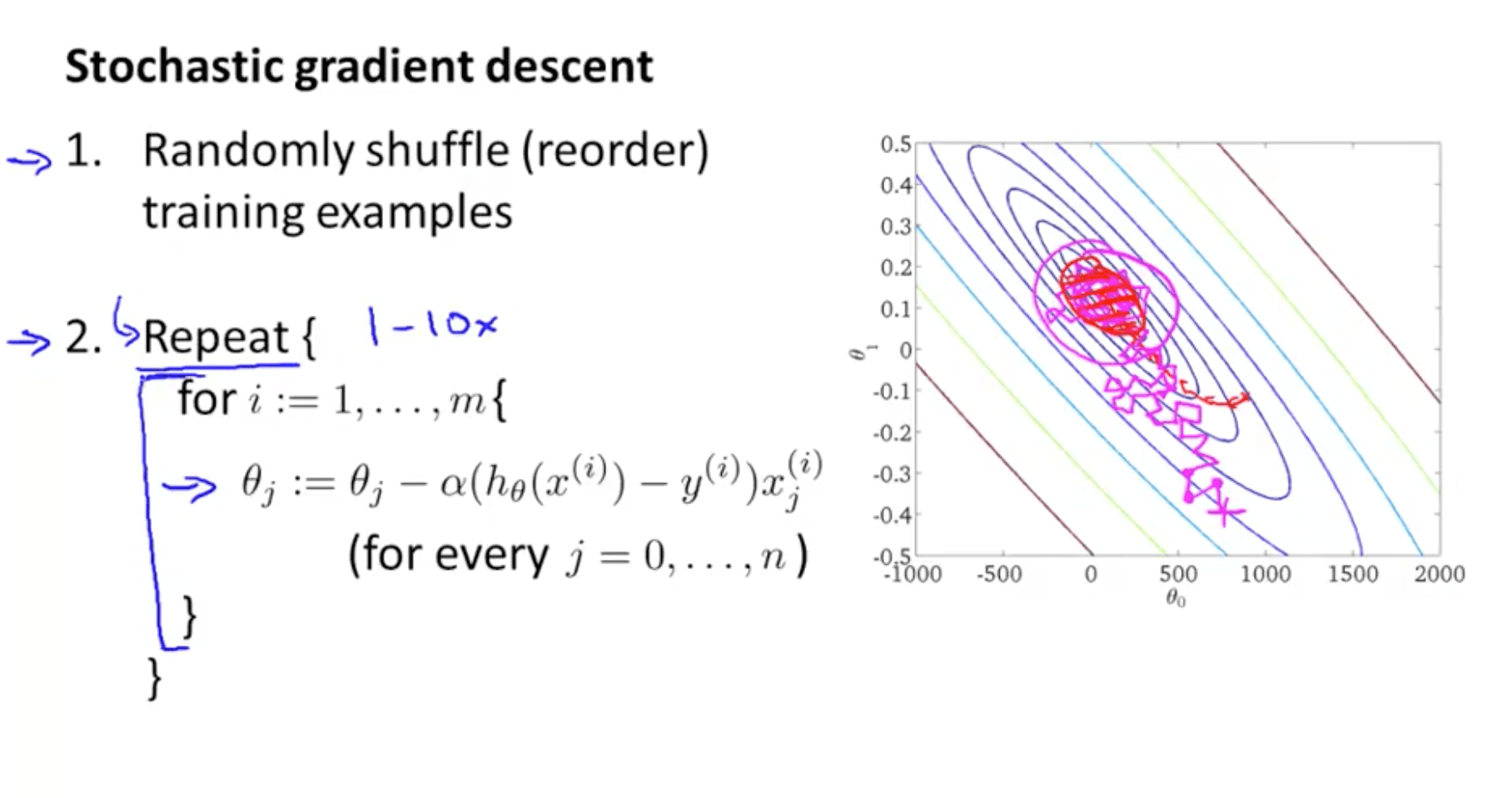• This may some times be faster than stochastic gradient descent
• Difference
• Use all m examples in each iteration
• Use 1 example in each iteration
• Use b examples in each iteration
• b = mini-batch size
• b = 10 examples
• After looking at the 10 training examples, we start making progress in modifying the parameters
• Mini-batch gradient descent can outperform stochastic gradient descent if we use a vectorized implementation
• This algorithm is the same as batch gradient descent if b = m, where we iterate across all training examples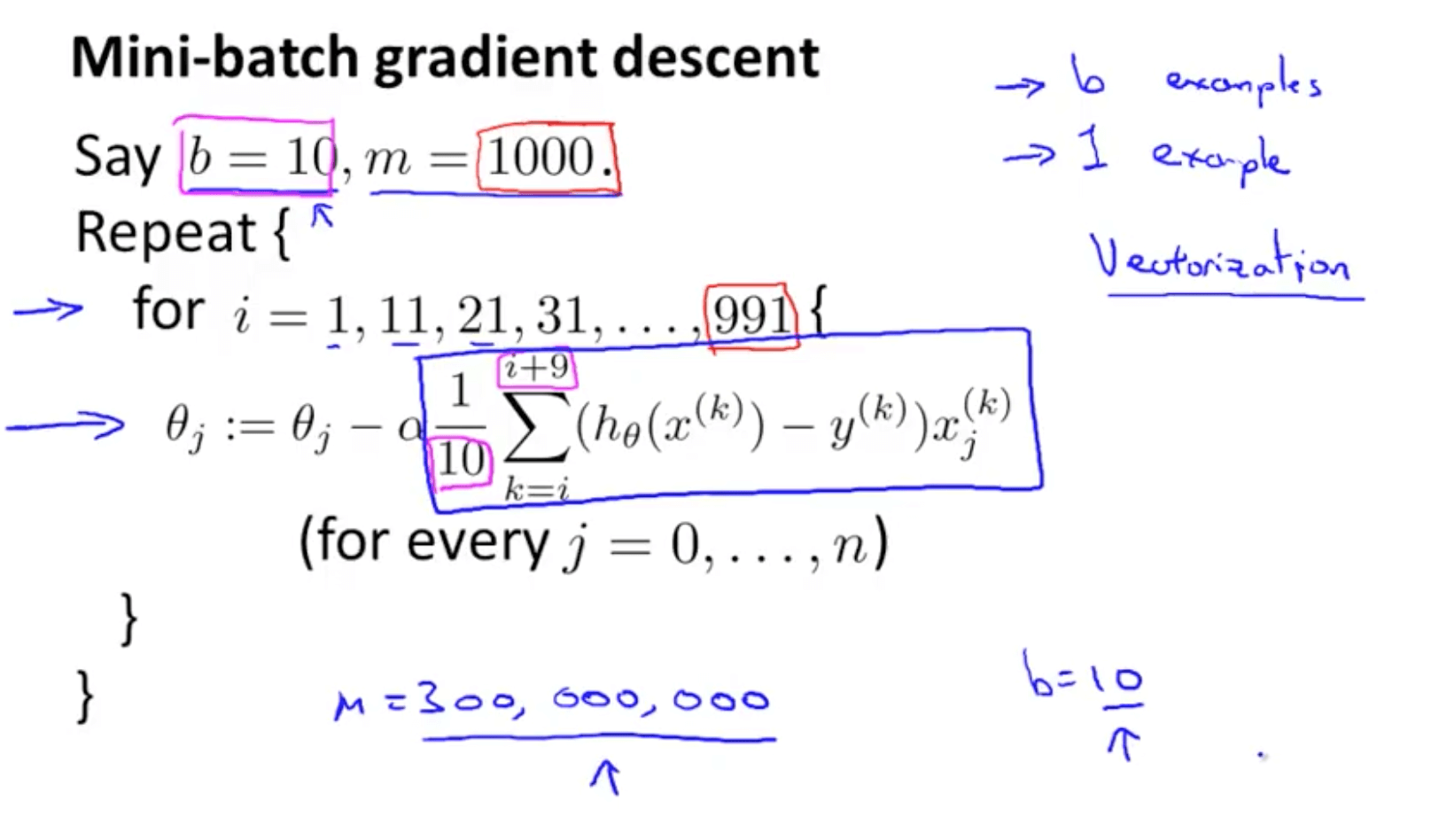### 1d. Stochastic Gradient Descent Convergence

• Checking for convergence
• When you average over a smaller number of examples, there might be too much noise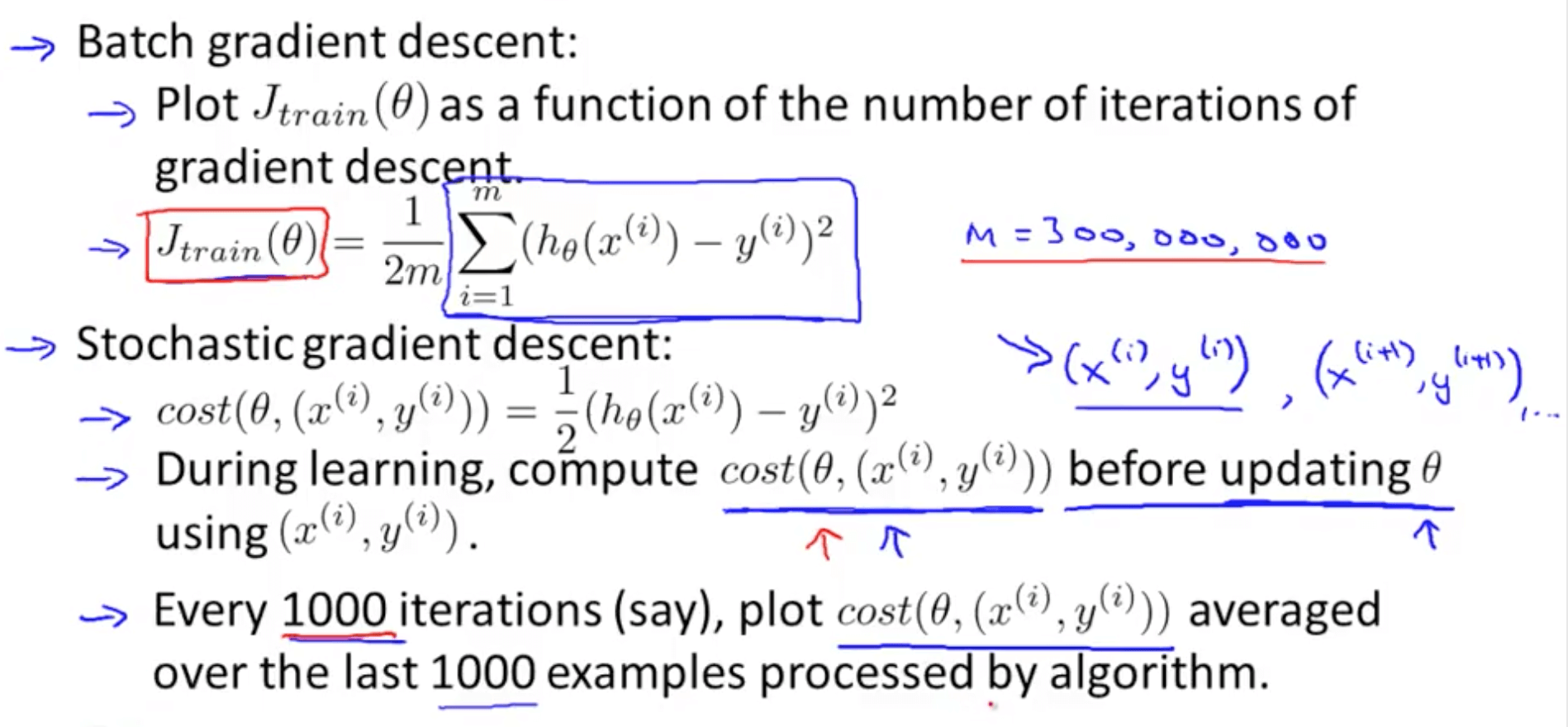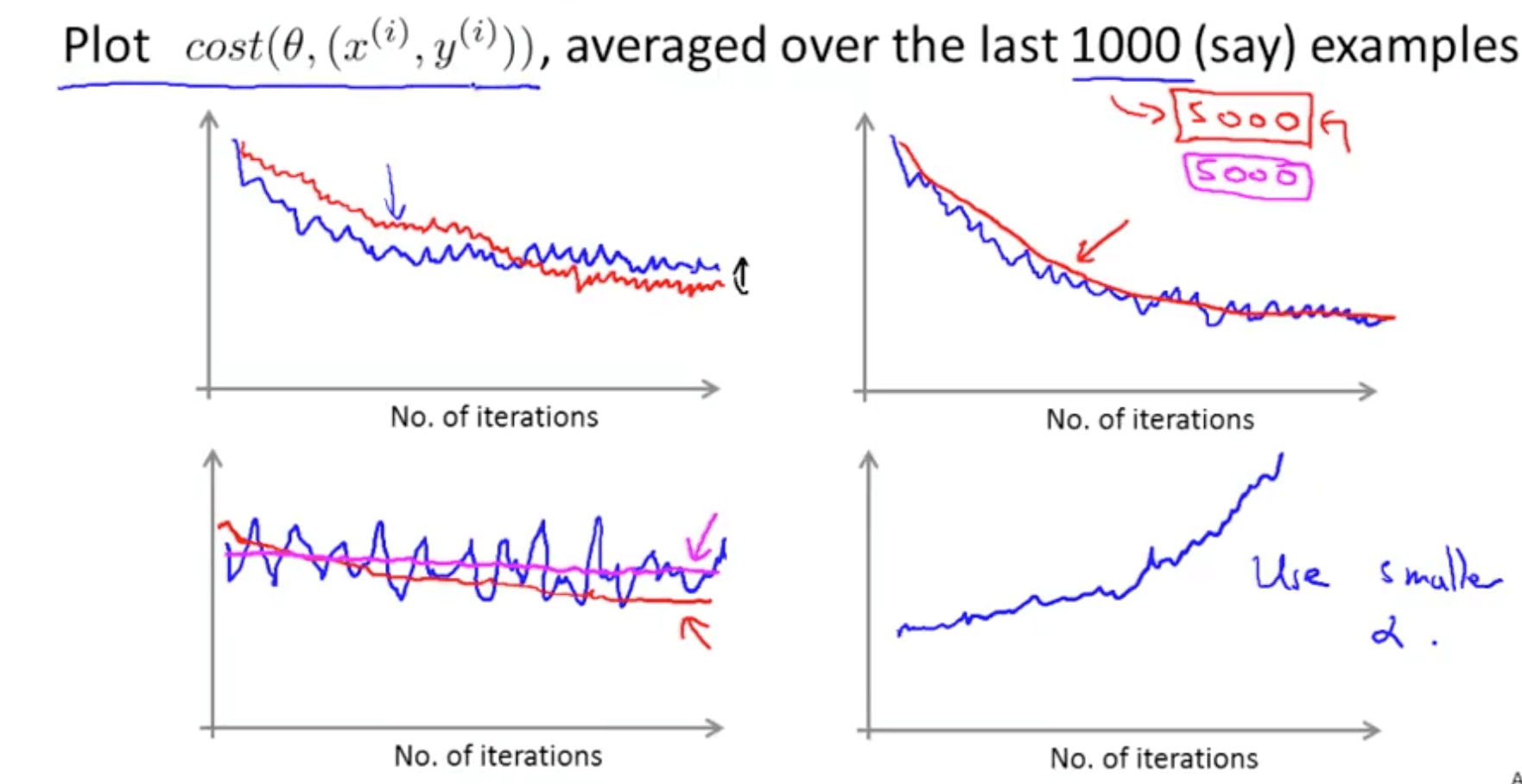• Stochastic gradient descent learning rate issue
• Decrease learning rate to allow convergence for a slightly better hypothesis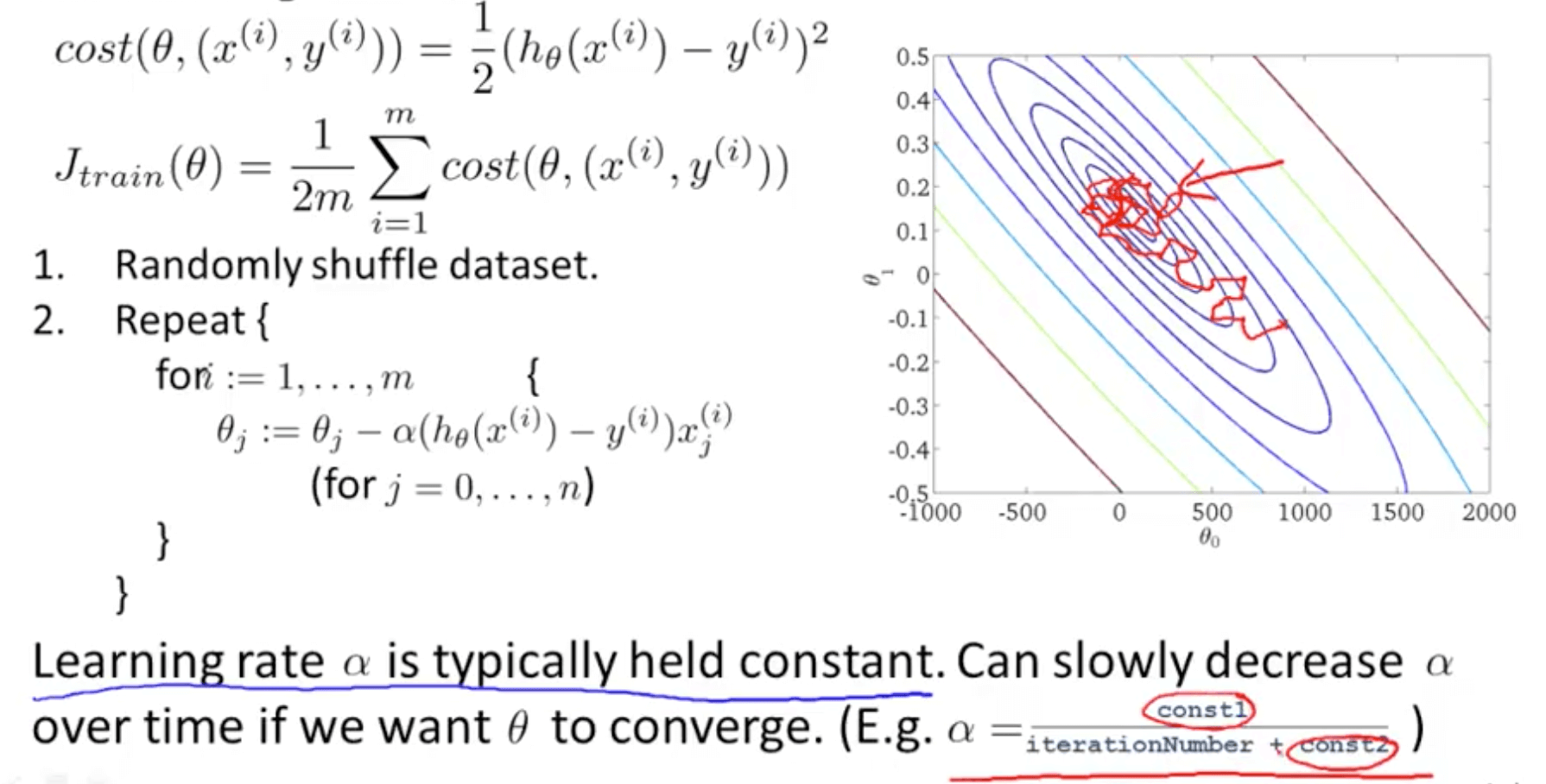### 2a. Online Learning

• What is online learning?
• When we have a continuous stream of information and we want to learn from that
• We can use an online learning algorithm to optimize some decisions
• Example of online learning: learning whether to buy or not buy
• Shipping service website where user comes, specifies origin and destination, you offer to ship their package for some asking price, and users sometimes choose to use your shipping service (y = 1), sometimes not (y = 0)
• Features x captures properties of user, of origin/destination and asking price
•  We want to learn p(y = 1 x;θ) to optimize price
• Probability of 1 given price and features
• We can use logistic regression or neural network
• Online learning algorithm
• If you have a large number of users, you can do this
• If you have a small number of users, you should save the data and train your parameters on your training data, not on a continuous stream of data
• This online algorithm can adapt to users’ preferences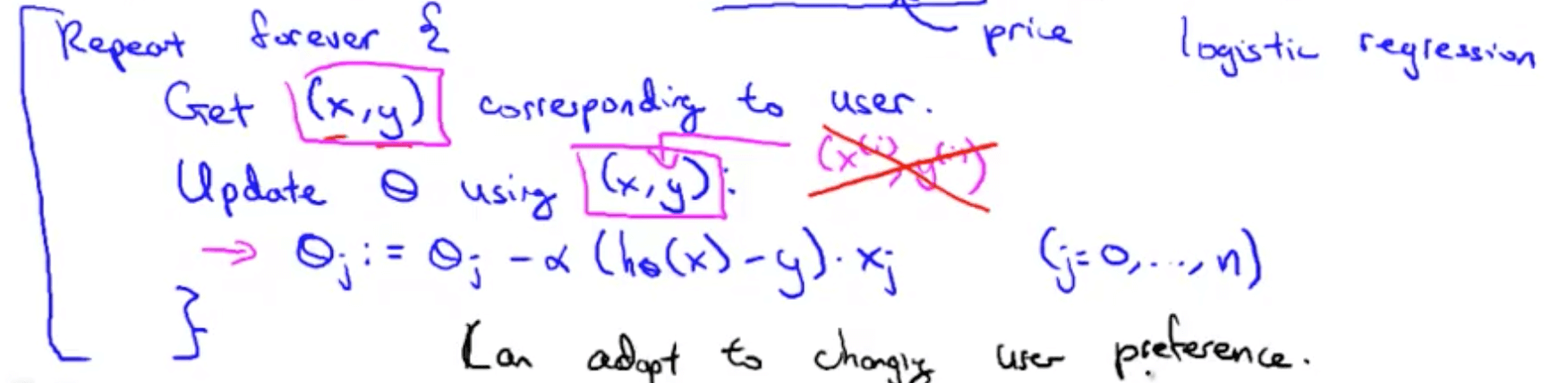• Example 2 of online learning: learning to search
• User searches for “Android phone 1080p camera”
• Have 100 phones in store with 10 results
• x = features of phone, how many words in user query match name of phone, how many words in query match description of phone
• y = 1 if user clicks on link
• y = 0 if otherwise
•  We want to learn p(y = 1 x;θ) to predict CTR
• Use to show users’ 10 phones they’re most likely to click on
• Other examples
• Choosing special offers to show user
• Customized selection of news articles
• Product recommendation

### 2b. Map Reduce and Data Parallelism

• Map reduce and linear regression
• This is an alternative to stochastic gradient descent and mini-batch gradient descent
• It is exactly equal to batch gradient descent but
1. Split the training sets
2. Split to 4 computers
3. Combine results
• Many learning algorithms can be expressed as computing sums of functions over the training set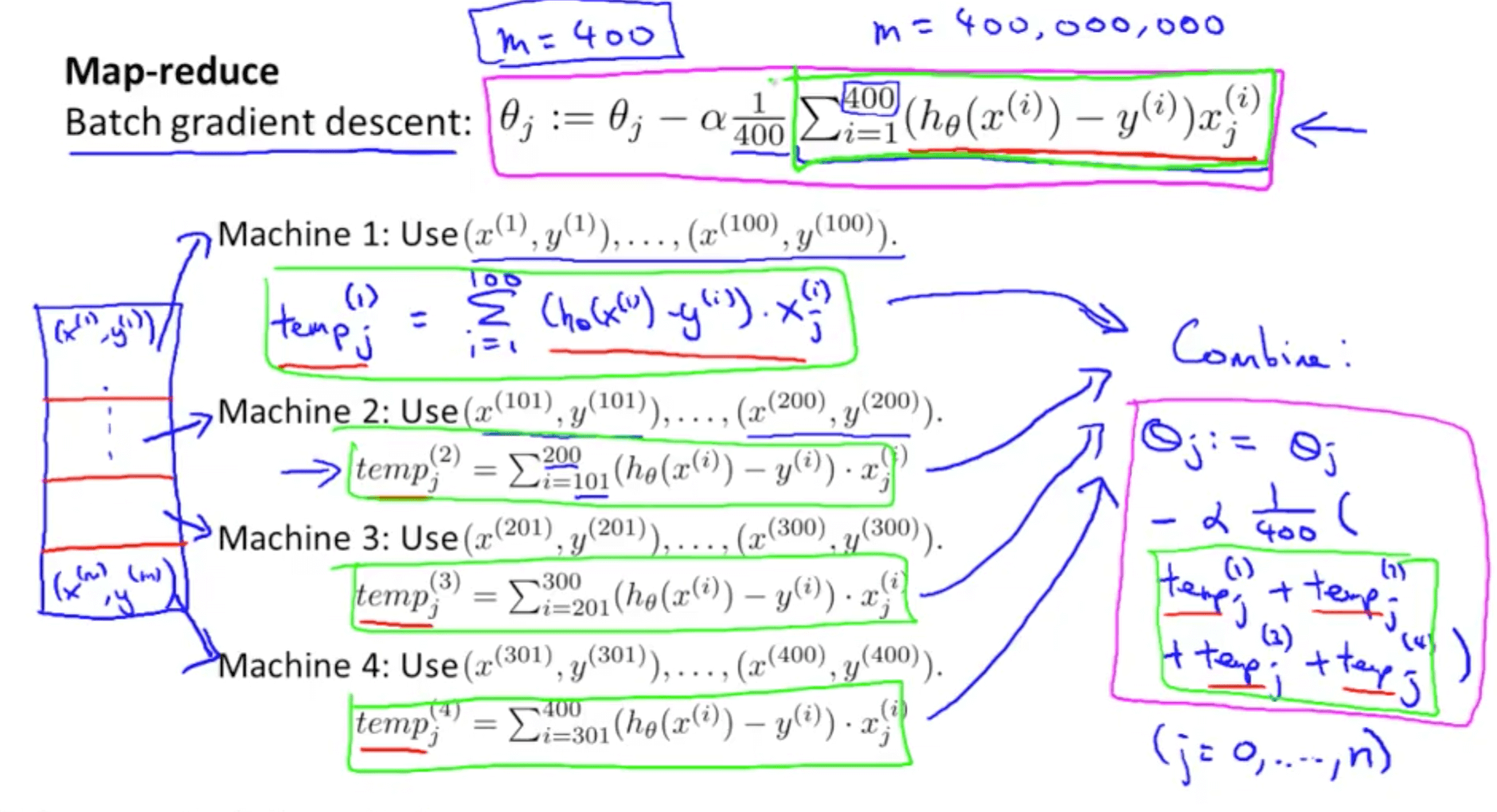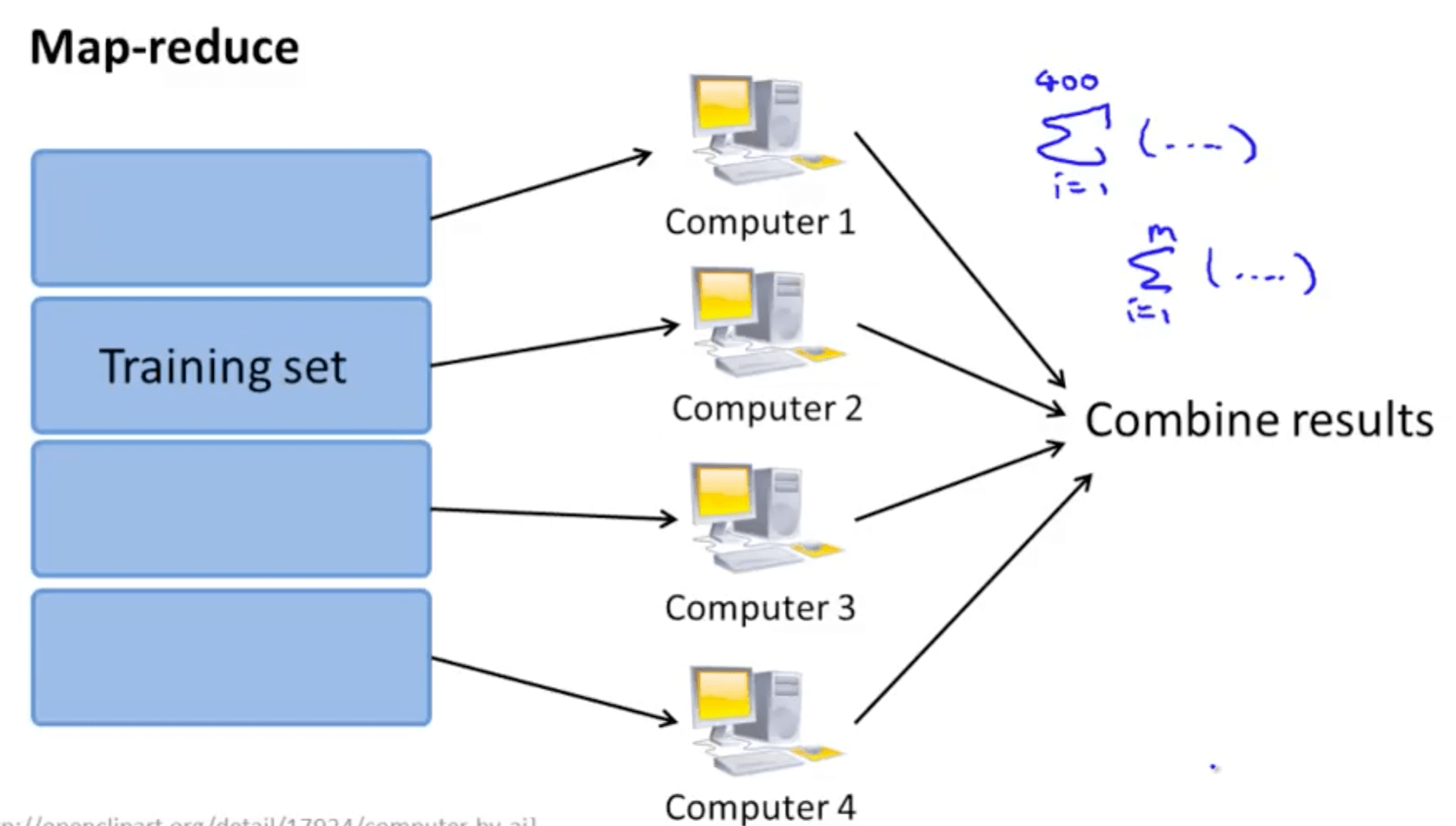• Map reduce and logistic regression
• This can be done too as we can compute sums of fractions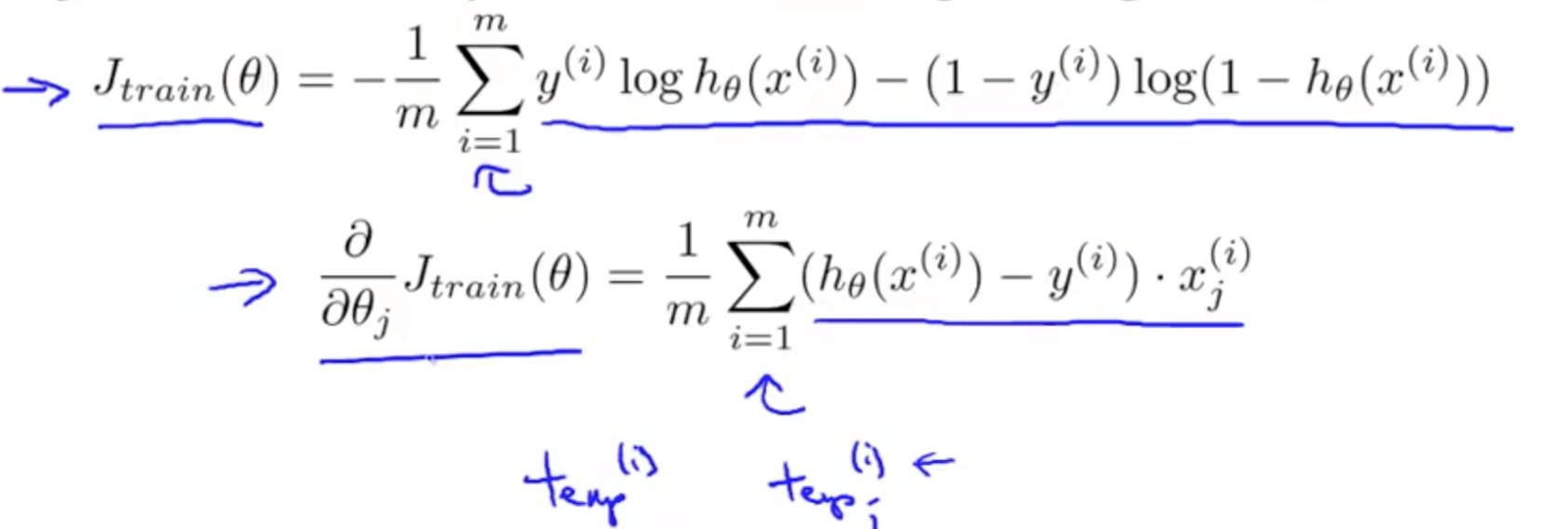• Map reduce and neural network
• Suppose you want to apply map-reduce to train a neural network on 10 machines
• In each iteration, compute forward propagation and back propagation on 1/10 of the data to compute the derivative with respect to that 1/10 of the data
• Multi-core machines
• We can split training sets to different cores and then combine the results
• “Parallelizing” over multiple cores in the same machine makes network latency less of an issue
• There are some libraries to automatically “parallelize” by just implementing the usual vectorized implementation
Tags: